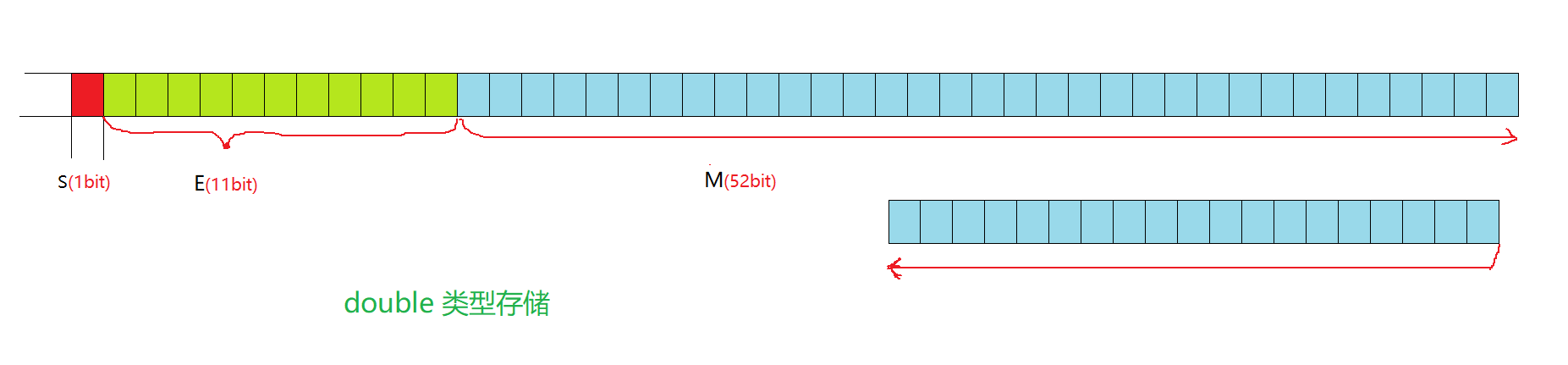﻿ C语言数据存储归类介绍_C 语言_脚本之家
C 语言# C语言数据存储归类介绍

## 数据类型的介绍

• 决定了访问内存空间的大小
• 决定了看待内存空间的视角（例如：整型和字符数据类型）

## 类型的基本归类

char
unsigned char
signed char
short
unsigned short [int]
signed short [int]
int
unsigned int
signed int
long
unsigned long [int]
signed long [int]

float
double

> 数组类型
> 结构体类型    struct
> 枚举类型    enum
> 联合类型    union

```int *pi;
char *pc;
float *pf;
void *pv;（泛型指针）
```

void 表示空类型 (无类型)

```//作为函数的返回类型
void f1()//不接受任何返回值
{
;
}
//作为函数的参数
int f2(void)//参数为void时，传入参数会报错
{
;
}
//作为指针类型
void* f3(void* pv)//参数为 void* 时，可接收任意类型的参数
{
;
}```

## 整型在内存中的存储## 浮点数在内存中的存储

```int main()
{
int n = 9;
float* pf = (float*)&n;
printf("n的值为：%d\n", n);
printf("pf的值为：%f\n", *pf);
*pf = 9.0;
printf("n的值为：%d\n", n);
printf("pf的值为：%f\n", *pf);
return 0;
}```## 浮点数在内存中的存储规则

• (-1)^S * M * 2^E
• (-1)^S 代表 符号位，S=0为正数，S=1为负数
• M 表示有效数字，（1 ≤ M < 2）
• 2^E表示指数位

IEEE 754规定：IEEE 754对 有效数字M 和 指数E 有一些特殊的规定：

0  10000001 01000000000000000000000
S     E           M

0100 0000 1010 0000 0000 0000 0000 0000

4    0     a    0    0    0    0    0

40 a0 00 00

00 00 a0 40• 符号为负 `S = 1`
• 小数点前正着写二进制，小数点后按照`2^(-n)` ，n = 4 (1/4=0.25)，（倒着写二进制），所以二进制为 `10.01`
• 用科学计数表示 `1.001 x 2^(1)`
• 指数E进行替换 `E = 1 + 127 = 128 = 1000 0000(二进制)`
• 由上可得 `S = 1` `E = 1000 0000` `M = 1.001`

1 10000000 00100000000000000000000
S    E             M

1100 0000 0001 0000 0000 0000 0000 0000

c     0    1    0    0    0    0     0

c0 10 00 00

00 00 10 c0E全为0：

E全为1：